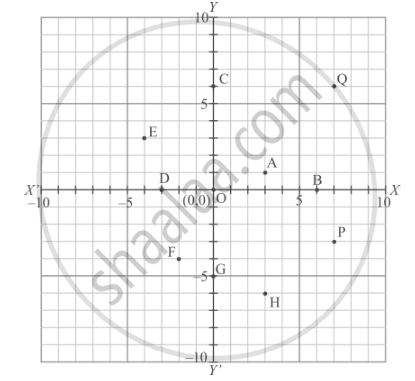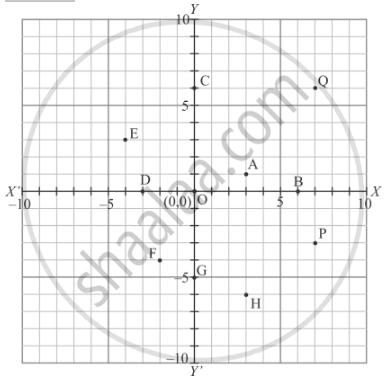# Write the Coordinates of Each of the Following Points Marked in the Graph Paper: - Mathematics

Write the coordinates of each of the following points marked in the graph paper:#### Solution

The following graph is given in the question with the marked points and we are asked to write down their coordinates.The distance of point A from y-axis is 3 units and that of from x-axis is 1 units. Since A lies in the first quadrant, so its coordinates are (3,1).

The distance of point B from y-axis is 6 units and that of from x-axis is 0 units. Since B lies on x-axis, so its coordinates are (6,0).

The distance of point C from y-axis is 0 units and that of from x-axis is 6 units. Since C lies on y-axis, so its coordinates are (0,6).

The distance of point D from y-axis is -3 units and that of from x-axis is 0 units. Since D lies on x-axis, so its coordinates are (-3 ,0).

The distance of point E from y-axis is -4 units and that of from x-axis is 3 units. Since E lies in the second quadrant, so its coordinates are (-4 , 3).

The distance of point F from y-axis is -2 units and that of from x-axis is -4 units. Since F lies in the third quadrant, so its coordinates are (-2 , -4).

The distance of point G from y-axis is 0 units and that of from x-axis is -5 units. Since G lies on y-axis, so its coordinates are (0,-5).

The distance of point H from y-axis is 3 units and that of from x-axis is -6 units. Since H lies in the fourth quadrant, so its coordinates are (3 , -6).

The distance of point P from y-axis is 7 units and that of from x-axis is -3 units. Since P lies in the fourth quadrant, so its coordinates are (7 , -3).

The distance of point Q from y-axis is 7 units and that of from x-axis is 6 units. Since Q lies in the first quadrant, so its coordinates are (7,6).

Concept: Plotting a Point in the Plane If Its Coordinates Are Given.
Is there an error in this question or solution?

#### APPEARS IN

RD Sharma Mathematics for Class 9
Chapter 8 Co-ordinate Geometry
Exercise 8.1 | Q 2 | Page 6

Share